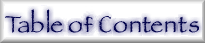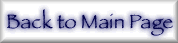## 13. Explanation of Tables and Tabulated Figures

The Tables follow a consistent grid. The figures are adapted to highlight the region of energy affected for each element, and hence vary with the atomic number.

NOTE: The symbols used here are slightly different from Chantler , in order to clearly differentiate the mass attenuation coefficients from the atomic cross-sections and the linear absorption coefficients, but the meanings are identical.

 Z Atomic number E Energy in keV λ Equivalent wavelength using E. λ = 1.239 842 44 keV - nm, from ref.  Atomic weight g/mol, used in determination of conversion factors ρ Nominal density in typical elemental material for determination of linear absorption coefficients µ = [µ/ρ]ρ τPE = σPE Atomic photoabsorption cross-section in barns/atom Edge energies Values taken from ref.  Edge labels Spectroscopic notation (K = 1s, LI = 2s, LII = 2p1/2, et seq.) f1, f2 Atomic form factors in eu (electrons/atom) for forward scattering following (eq 4) and (eq 7). frel Relativistic correction to Re(f), following ref.  (denoted H82), and refs. [22-25], scaled as in refs. [16,17],  (denoted 3/5CL) fNT Nuclear Thomson correction to Re(f) following (eq 4) and ref. [10,11] f2, K-shell Component of f2 relating to the isolated K-shell orbital [µ/ρ]PE = σPE/uA Mass photoelectric attenuation coefficient (cm2/g) [µ/ρ]PE,K Component of [µ/ρ]PE relating to the isolated K-shell orbital (cm2/g) σ/ρ (coh+inc) Estimate of coherent and incoherent (σcoh + σincoh) scattering cross-section sum (cm2/g) [µ/ρ]tot = σtot/uA Mass attenuation coefficient (cm2/g) (eq 8) Full lines on plots Current theoretical tabulation for f1, f2 + Earlier theoretical implementation  showing limited convergence near selected edges Thick dashes Henke et al., experimental synthesis (ref. [32,33]) Dotted curves with crosses Theoretical results for comparison reinterpolated from ref. [28,29], , for f2 and [µ/ρ] Circles with error bars Experimental results, summarized in ref.